# Mean State

Period Mean (original grids) [Watt m-2]
Model Period Mean (intersection) [Watt m-2]
Model Period Mean (complement) [Watt m-2]
Benchmark Period Mean (intersection) [Watt m-2]
Benchmark Period Mean (complement) [Watt m-2]
Bias [Watt m-2]
RMSE [Watt m-2]
Phase Shift [months]
Bias Score 
RMSE Score 
Seasonal Cycle Score 
Spatial Distribution Score 
Interannual Variability Score 
Overall Score 
Benchmark [-] 453.
CRUNCEPv7 [-] 460. 460. 453. 460. 7.88 14.3 1.69 0.400 0.393 0.748 0.997 0.715 0.608
GSWP3v1 [-] 459. 459. 453. 460. 6.81 14.2 1.66 0.386 0.378 0.756 0.970 0.709 0.596
WFDEI [-] 461. 461. 453. 460. 8.70 16.5 1.81 0.309 0.360 0.725 0.991 0.708 0.575
Period Mean (original grids) [Watt m-2]
Model Period Mean (intersection) [Watt m-2]
Model Period Mean (complement) [Watt m-2]
Benchmark Period Mean (intersection) [Watt m-2]
Benchmark Period Mean (complement) [Watt m-2]
Bias [Watt m-2]
RMSE [Watt m-2]
Phase Shift [months]
Bias Score 
RMSE Score 
Seasonal Cycle Score 
Spatial Distribution Score 
Interannual Variability Score 
Overall Score 
Benchmark [-] 471.
CRUNCEPv7 [-] 479. 479. 471. 456. 7.66 19.5 0.787 0.603 0.502 0.940 0.999 0.737 0.714
GSWP3v1 [-] 476. 476. 471. 456. 4.51 17.3 0.653 0.671 0.517 0.952 0.972 0.694 0.721
WFDEI [-] 476. 477. 471. 456. 5.04 20.9 0.873 0.635 0.455 0.927 0.999 0.714 0.698
Period Mean (original grids) [Watt m-2]
Model Period Mean (intersection) [Watt m-2]
Model Period Mean (complement) [Watt m-2]
Benchmark Period Mean (intersection) [Watt m-2]
Benchmark Period Mean (complement) [Watt m-2]
Bias [Watt m-2]
RMSE [Watt m-2]
Phase Shift [months]
Bias Score 
RMSE Score 
Seasonal Cycle Score 
Spatial Distribution Score 
Interannual Variability Score 
Overall Score 
Benchmark [-] 443.
CRUNCEPv7 [-] 446. 446. 443. 444. 3.27 15.5 0.804 0.623 0.540 0.908 0.983 0.626 0.703
GSWP3v1 [-] 446. 446. 443. 444. 2.70 14.7 0.880 0.671 0.526 0.893 1.00 0.612 0.705
WFDEI [-] 445. 445. 443. 444. 1.96 17.5 1.28 0.645 0.466 0.828 0.989 0.591 0.664
Period Mean (original grids) [Watt m-2]
Model Period Mean (intersection) [Watt m-2]
Model Period Mean (complement) [Watt m-2]
Benchmark Period Mean (intersection) [Watt m-2]
Benchmark Period Mean (complement) [Watt m-2]
Bias [Watt m-2]
RMSE [Watt m-2]
Phase Shift [months]
Bias Score 
RMSE Score 
Seasonal Cycle Score 
Spatial Distribution Score 
Interannual Variability Score 
Overall Score 
Benchmark [-] 276.
CRUNCEPv7 [-] 264. 264. 275. 292. -8.66 19.3 0.0696 0.854 0.776 0.995 0.970 0.763 0.856
GSWP3v1 [-] 275. 275. 275. 292. 3.25 15.4 0.0642 0.906 0.805 0.996 0.990 0.794 0.882
WFDEI [-] 261. 261. 275. 292. -11.6 22.8 0.0885 0.825 0.742 0.994 0.987 0.797 0.848
Period Mean (original grids) [Watt m-2]
Model Period Mean (intersection) [Watt m-2]
Model Period Mean (complement) [Watt m-2]
Benchmark Period Mean (intersection) [Watt m-2]
Benchmark Period Mean (complement) [Watt m-2]
Bias [Watt m-2]
RMSE [Watt m-2]
Phase Shift [months]
Bias Score 
RMSE Score 
Seasonal Cycle Score 
Spatial Distribution Score 
Interannual Variability Score 
Overall Score 
Benchmark [-] 456.
CRUNCEPv7 [-] 455. 455. 453. 465. 3.63 9.59 2.03 0.334 0.398 0.682 0.999 0.660 0.579
GSWP3v1 [-] 456. 456. 453. 465. 4.24 9.34 1.81 0.340 0.419 0.719 0.897 0.705 0.583
WFDEI [-] 459. 459. 453. 465. 7.14 11.6 2.12 0.275 0.358 0.670 0.991 0.738 0.565
Period Mean (original grids) [Watt m-2]
Model Period Mean (intersection) [Watt m-2]
Model Period Mean (complement) [Watt m-2]
Benchmark Period Mean (intersection) [Watt m-2]
Benchmark Period Mean (complement) [Watt m-2]
Bias [Watt m-2]
RMSE [Watt m-2]
Phase Shift [months]
Bias Score 
RMSE Score 
Seasonal Cycle Score 
Spatial Distribution Score 
Interannual Variability Score 
Overall Score 
Benchmark [-] 306.
CRUNCEPv7 [-] 309. 309. 306. 329. 3.75 11.7 0.0220 0.918 0.868 0.999 0.970 0.888 0.918
GSWP3v1 [-] 315. 315. 306. 329. 9.60 14.5 0.0208 0.865 0.862 0.999 0.993 0.891 0.912
WFDEI [-] 306. 305. 306. 329. 0.0507 16.1 0.0229 0.940 0.799 0.998 0.983 0.866 0.898
Period Mean (original grids) [Watt m-2]
Model Period Mean (intersection) [Watt m-2]
Model Period Mean (complement) [Watt m-2]
Benchmark Period Mean (intersection) [Watt m-2]
Benchmark Period Mean (complement) [Watt m-2]
Bias [Watt m-2]
RMSE [Watt m-2]
Phase Shift [months]
Bias Score 
RMSE Score 
Seasonal Cycle Score 
Spatial Distribution Score 
Interannual Variability Score 
Overall Score 
Benchmark [-] 362.
CRUNCEPv7 [-] 361. 360. 361. 395. 2.02 14.2 0.109 0.867 0.832 0.992 0.999 0.746 0.878
GSWP3v1 [-] 364. 364. 361. 395. 5.28 15.5 0.105 0.853 0.822 0.992 0.986 0.723 0.866
WFDEI [-] 359. 358. 361. 395. -0.0312 19.0 0.121 0.858 0.766 0.991 0.995 0.735 0.852
Period Mean (original grids) [Watt m-2]
Model Period Mean (intersection) [Watt m-2]
Model Period Mean (complement) [Watt m-2]
Benchmark Period Mean (intersection) [Watt m-2]
Benchmark Period Mean (complement) [Watt m-2]
Bias [Watt m-2]
RMSE [Watt m-2]
Phase Shift [months]
Bias Score 
RMSE Score 
Seasonal Cycle Score 
Spatial Distribution Score 
Interannual Variability Score 
Overall Score 
Benchmark [-] 450.
CRUNCEPv7 [-] 454. 454. 450. 463. 6.72 17.8 0.511 0.617 0.579 0.956 0.992 0.639 0.727
GSWP3v1 [-] 449. 449. 450. 463. 2.04 16.5 0.646 0.686 0.566 0.936 0.996 0.622 0.729
WFDEI [-] 451. 452. 450. 463. 4.86 19.2 0.611 0.628 0.521 0.943 0.999 0.624 0.706
Period Mean (original grids) [Watt m-2]
Model Period Mean (intersection) [Watt m-2]
Model Period Mean (complement) [Watt m-2]
Benchmark Period Mean (intersection) [Watt m-2]
Benchmark Period Mean (complement) [Watt m-2]
Bias [Watt m-2]
RMSE [Watt m-2]
Phase Shift [months]
Bias Score 
RMSE Score 
Seasonal Cycle Score 
Spatial Distribution Score 
Interannual Variability Score 
Overall Score 
Benchmark [-] 275.
CRUNCEPv7 [-] 271. 271. 275. 280. -3.37 15.2 0.0550 0.913 0.794 0.996 0.971 0.849 0.886
GSWP3v1 [-] 281. 281. 275. 280. 7.26 14.4 0.0339 0.883 0.825 0.997 0.993 0.847 0.895
WFDEI [-] 269. 269. 275. 280. -5.14 19.9 0.0466 0.899 0.737 0.996 0.986 0.873 0.871
Period Mean (original grids) [Watt m-2]
Model Period Mean (intersection) [Watt m-2]
Model Period Mean (complement) [Watt m-2]
Benchmark Period Mean (intersection) [Watt m-2]
Benchmark Period Mean (complement) [Watt m-2]
Bias [Watt m-2]
RMSE [Watt m-2]
Phase Shift [months]
Bias Score 
RMSE Score 
Seasonal Cycle Score 
Spatial Distribution Score 
Interannual Variability Score 
Overall Score 
Benchmark [-] 448.
CRUNCEPv7 [-] 446. 446. 450. 423. -6.18 14.9 0.346 0.766 0.716 0.971 0.994 0.719 0.814
GSWP3v1 [-] 446. 446. 450. 423. -6.14 15.4 0.362 0.769 0.709 0.971 0.982 0.692 0.805
WFDEI [-] 444. 444. 450. 423. -7.75 19.8 0.428 0.735 0.624 0.965 0.992 0.673 0.769
Period Mean (original grids) [Watt m-2]
Model Period Mean (intersection) [Watt m-2]
Model Period Mean (complement) [Watt m-2]
Benchmark Period Mean (intersection) [Watt m-2]
Benchmark Period Mean (complement) [Watt m-2]
Bias [Watt m-2]
RMSE [Watt m-2]
Phase Shift [months]
Bias Score 
RMSE Score 
Seasonal Cycle Score 
Spatial Distribution Score 
Interannual Variability Score 
Overall Score 
Benchmark [-] 364.
CRUNCEPv7 [-] 361. 361. 362. 385. 1.87 10.4 0.204 0.859 0.817 0.986 1.00 0.822 0.884
GSWP3v1 [-] 362. 362. 362. 385. 3.36 10.7 0.231 0.860 0.811 0.984 0.990 0.825 0.880
WFDEI [-] 355. 355. 362. 385. -3.92 15.5 0.232 0.855 0.707 0.984 0.998 0.824 0.846
Period Mean (original grids) [Watt m-2]
Model Period Mean (intersection) [Watt m-2]
Model Period Mean (complement) [Watt m-2]
Benchmark Period Mean (intersection) [Watt m-2]
Benchmark Period Mean (complement) [Watt m-2]
Bias [Watt m-2]
RMSE [Watt m-2]
Phase Shift [months]
Bias Score 
RMSE Score 
Seasonal Cycle Score 
Spatial Distribution Score 
Interannual Variability Score 
Overall Score 
Benchmark [-] 397.
CRUNCEPv7 [-] 376. 376. 376. 406. 0.929 15.5 0.478 0.727 0.676 0.947 0.999 0.696 0.787
GSWP3v1 [-] 378. 378. 376. 406. 3.49 15.7 0.471 0.718 0.690 0.948 0.998 0.735 0.797
WFDEI [-] 375. 375. 376. 406. -0.437 18.8 0.542 0.711 0.624 0.936 0.998 0.739 0.772
Period Mean (original grids) [Watt m-2]
Model Period Mean (intersection) [Watt m-2]
Model Period Mean (complement) [Watt m-2]
Benchmark Period Mean (intersection) [Watt m-2]
Benchmark Period Mean (complement) [Watt m-2]
Bias [Watt m-2]
RMSE [Watt m-2]
Phase Shift [months]
Bias Score 
RMSE Score 
Seasonal Cycle Score 
Spatial Distribution Score 
Interannual Variability Score 
Overall Score 
Benchmark [-] 464.
CRUNCEPv7 [-] 454. 454. 465. 441. -11.0 21.2 0.412 0.748 0.743 0.967 0.994 0.646 0.807
GSWP3v1 [-] 450. 450. 465. 441. -15.3 24.0 0.413 0.710 0.732 0.967 0.970 0.644 0.793
WFDEI [-] 447. 447. 465. 441. -17.8 28.7 0.407 0.667 0.687 0.963 0.966 0.620 0.765
Period Mean (original grids) [Watt m-2]
Model Period Mean (intersection) [Watt m-2]
Model Period Mean (complement) [Watt m-2]
Benchmark Period Mean (intersection) [Watt m-2]
Benchmark Period Mean (complement) [Watt m-2]
Bias [Watt m-2]
RMSE [Watt m-2]
Phase Shift [months]
Bias Score 
RMSE Score 
Seasonal Cycle Score 
Spatial Distribution Score 
Interannual Variability Score 
Overall Score 
Benchmark [-] 278.
CRUNCEPv7 [-] 277. 276. 277. 289. -1.62 14.4 0.0681 0.896 0.805 0.995 0.964 0.775 0.873
GSWP3v1 [-] 285. 285. 277. 289. 8.61 14.5 0.0560 0.855 0.833 0.996 0.993 0.815 0.887
WFDEI [-] 273. 273. 277. 289. -4.48 18.5 0.0897 0.880 0.751 0.994 0.964 0.855 0.866
Period Mean (original grids) [Watt m-2]
Model Period Mean (intersection) [Watt m-2]
Model Period Mean (complement) [Watt m-2]
Benchmark Period Mean (intersection) [Watt m-2]
Benchmark Period Mean (complement) [Watt m-2]
Bias [Watt m-2]
RMSE [Watt m-2]
Phase Shift [months]
Bias Score 
RMSE Score 
Seasonal Cycle Score 
Spatial Distribution Score 
Interannual Variability Score 
Overall Score 
Benchmark [-] 444.
CRUNCEPv7 [-] 441. 441. 442. 455. 3.80 13.8 0.559 0.562 0.603 0.943 0.918 0.723 0.725
GSWP3v1 [-] 443. 442. 442. 455. 4.69 12.6 0.636 0.602 0.605 0.936 0.982 0.721 0.742
WFDEI [-] 445. 445. 442. 455. 6.76 16.1 0.653 0.542 0.531 0.931 0.965 0.714 0.703
Period Mean (original grids) [Watt m-2]
Model Period Mean (intersection) [Watt m-2]
Model Period Mean (complement) [Watt m-2]
Benchmark Period Mean (intersection) [Watt m-2]
Benchmark Period Mean (complement) [Watt m-2]
Bias [Watt m-2]
RMSE [Watt m-2]
Phase Shift [months]
Bias Score 
RMSE Score 
Seasonal Cycle Score 
Spatial Distribution Score 
Interannual Variability Score 
Overall Score 
Benchmark [-] 386.
CRUNCEPv7 [-] 387. 387. 384. 429. 5.67 9.79 0.109 0.867 0.862 0.993 0.999 0.887 0.911
GSWP3v1 [-] 389. 389. 384. 429. 7.56 10.8 0.117 0.845 0.864 0.992 0.998 0.864 0.905
WFDEI [-] 384. 384. 384. 429. 2.37 14.7 0.128 0.898 0.745 0.991 0.999 0.892 0.878
Period Mean (original grids) [Watt m-2]
Model Period Mean (intersection) [Watt m-2]
Model Period Mean (complement) [Watt m-2]
Benchmark Period Mean (intersection) [Watt m-2]
Benchmark Period Mean (complement) [Watt m-2]
Bias [Watt m-2]
RMSE [Watt m-2]
Phase Shift [months]
Bias Score 
RMSE Score 
Seasonal Cycle Score 
Spatial Distribution Score 
Interannual Variability Score 
Overall Score 
Benchmark [-] 319.
CRUNCEPv7 [-] 323. 323. 318. 343. 5.76 12.2 0.0909 0.884 0.840 0.994 0.998 0.839 0.899
GSWP3v1 [-] 328. 328. 318. 343. 11.0 15.1 0.0824 0.821 0.839 0.994 0.987 0.836 0.886
WFDEI [-] 320. 320. 318. 343. 2.89 17.0 0.102 0.905 0.753 0.993 0.999 0.883 0.881
Period Mean (original grids) [Watt m-2]
Model Period Mean (intersection) [Watt m-2]
Model Period Mean (complement) [Watt m-2]
Benchmark Period Mean (intersection) [Watt m-2]
Benchmark Period Mean (complement) [Watt m-2]
Bias [Watt m-2]
RMSE [Watt m-2]
Phase Shift [months]
Bias Score 
RMSE Score 
Seasonal Cycle Score 
Spatial Distribution Score 
Interannual Variability Score 
Overall Score 
Benchmark [-] 376.
CRUNCEPv7 [-] 376. 377. 376. 379. 0.995 14.7 0.0948 0.866 0.813 0.994 0.998 0.755 0.873
GSWP3v1 [-] 379. 380. 376. 379. 4.24 16.3 0.0943 0.860 0.795 0.994 0.978 0.701 0.854
WFDEI [-] 376. 377. 376. 379. 1.59 19.2 0.0982 0.874 0.738 0.993 0.997 0.782 0.854
Period Mean (original grids) [Watt m-2]
Model Period Mean (intersection) [Watt m-2]
Model Period Mean (complement) [Watt m-2]
Benchmark Period Mean (intersection) [Watt m-2]
Benchmark Period Mean (complement) [Watt m-2]
Bias [Watt m-2]
RMSE [Watt m-2]
Phase Shift [months]
Bias Score 
RMSE Score 
Seasonal Cycle Score 
Spatial Distribution Score 
Interannual Variability Score 
Overall Score 
Benchmark [-] 449.
CRUNCEPv7 [-] 455. 455. 449. 458. 6.53 13.2 1.25 0.445 0.405 0.815 0.877 0.678 0.604
GSWP3v1 [-] 456. 456. 449. 458. 6.96 12.7 1.27 0.458 0.397 0.820 0.962 0.627 0.610
WFDEI [-] 457. 457. 449. 458. 8.73 14.8 1.64 0.394 0.366 0.752 0.907 0.665 0.575
Period Mean (original grids) [Watt m-2]
Model Period Mean (intersection) [Watt m-2]
Model Period Mean (complement) [Watt m-2]
Benchmark Period Mean (intersection) [Watt m-2]
Benchmark Period Mean (complement) [Watt m-2]
Bias [Watt m-2]
RMSE [Watt m-2]
Phase Shift [months]
Bias Score 
RMSE Score 
Seasonal Cycle Score 
Spatial Distribution Score 
Interannual Variability Score 
Overall Score 
Benchmark [-] 440.
CRUNCEPv7 [-] 446. 445. 440. 430. 4.53 11.0 0.664 0.711 0.629 0.922 0.997 0.750 0.773
GSWP3v1 [-] 446. 445. 440. 430. 4.67 10.9 0.682 0.731 0.616 0.915 0.999 0.716 0.766
WFDEI [-] 443. 443. 440. 430. 2.28 13.2 0.825 0.751 0.533 0.897 0.999 0.727 0.740
Period Mean (original grids) [Watt m-2]
Model Period Mean (intersection) [Watt m-2]
Model Period Mean (complement) [Watt m-2]
Benchmark Period Mean (intersection) [Watt m-2]
Benchmark Period Mean (complement) [Watt m-2]
Bias [Watt m-2]
RMSE [Watt m-2]
Phase Shift [months]
Bias Score 
RMSE Score 
Seasonal Cycle Score 
Spatial Distribution Score 
Interannual Variability Score 
Overall Score 
Benchmark [-] 387.
CRUNCEPv7 [-] 383. 382. 386. 394. -2.97 17.9 0.645 0.624 0.624 0.928 0.998 0.697 0.749
GSWP3v1 [-] 388. 387. 386. 394. 1.41 16.8 0.583 0.623 0.628 0.940 0.992 0.693 0.751
WFDEI [-] 389. 388. 386. 394. 3.08 18.9 0.597 0.614 0.568 0.940 0.999 0.733 0.737

# Temporally integrated period mean

BENCHMARK MEAN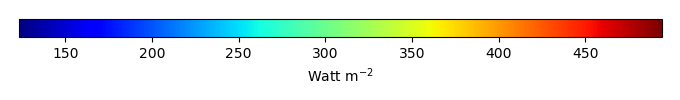MODEL MEANBIAS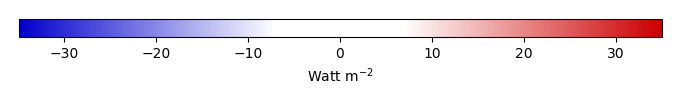BIAS SCORERMSE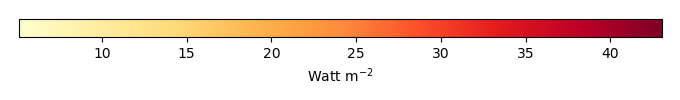RMSE SCOREBENCHMARK INTERANNUAL VARIABILITY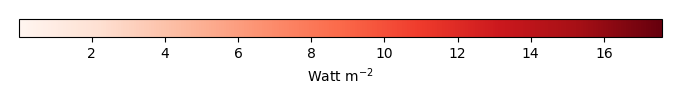MODEL INTERANNUAL VARIABILITYINTERANNUAL VARIABILITY SCOREBENCHMARK MAX MONTHMODEL MAX MONTHDIFFERENCE IN MAX MONTH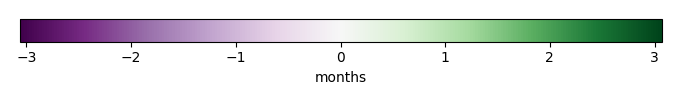SEASONAL CYCLE SCORESPATIAL TAYLOR DIAGRAMMODEL COLORS# Spatially integrated regional mean

MODEL COLORSREGIONAL MEANANNUAL CYCLEMONTHLY ANOMALYANNUAL CYCLE# All Models

BenchmarkCRUNCEPv7GSWP3v1WFDEI# Data Information

creation_date: Mon Jun 30 23:35:20 PDT 2014

source_file: This product is generated from monthly 1 degree GEWEX SRB Radiation observations